## Running remote host Weka experiments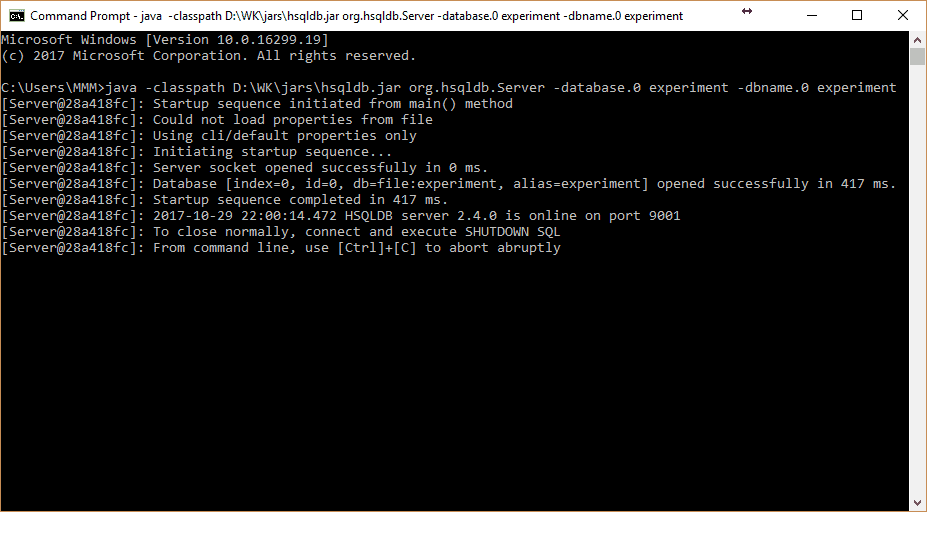Previously, we tried to run a weka server to utilize all cores of the processor in classification tasks. But it appears that the weka server works only in explorer for classification routines. For more advanced machine learning, there is a more flexible tool – experimenter. Weka server doesn’t support this area. So what to do if you want more performance or utilize the multi-core processor of the local machine. There is a way out, but it is quite tricky. Weka has the ability to perform remote experiments that allow spreading the load across multiple host machines that have Weka set up. You can read the documentation of remote experiments here, but it may be somewhat confusing. It took time for me to figure out some parts by trial and error. The trickiest part is to set everything up and prepare the necessary command to be run before performing a remote experiment. So let’s get to it.

## Utilizing multi-core processor for classification in WEKA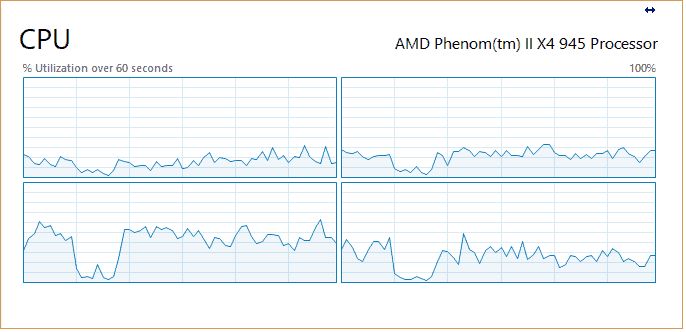Currently, WEKA is one of the most favorites machine learning tools. Without programming skills, you can do serious classification, clustering, and big data analysis. For some time I’ve been using its standard GUI features without thinking much about performance bottlenecks. But since researches are becoming more complex by using ensemble, voting and other meta-algorithms that generally are based on multiple classifiers running simultaneously, the performance issues start becoming annoying. You need to wait for hours until the task is completed. The problem is that when running classification algorithms from the WEKA GUI, the utilize a single core of your processor. Such algorithms as Multi-layer Percepron running 10 fold cross-validation is calculating one cross fold at the time on one core taking a long time to accomplish: So I started looking for options to make it use all cores of the processor as separate threads for each fold of operation. There are a couple of options available to do so. One is to use WekaServer package, and another is remote host processing. This time we will focus on WekaServer solution. The idea is to start a WEKA server as a distributed execution environment. When starting the server, you can indicate how…

## Implementing logistic regression learner with python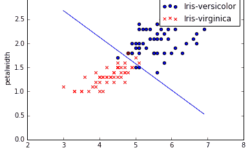Logistic regression is the next step from linear regression. The most real-life data have a non-linear relationship, thus applying linear models might be ineffective. Logistic regression is capable of handling non-linear effects in prediction tasks. You can think of lots of different scenarios where logistic regression could be applied. There can be financial, demographic, health, weather and other data where the model could be implemented and used to predict next events on future data. For instance, you can classify emails into spam and non-spam, transactions being fraud or not, tumors being malignant or benign. In order to understand logistic regression, let’s cover some basics, do a simple classification on data set with two features and then test it on real-life data with multiple features.

## Future of Artificial Intelligence; Its Implementations and LimitsArtificial Intelligence (AI) in computer science uses mechanical and computational processes to echo almost all aspects of human intelligence. AI can perform multiple functions: sensory interaction with the environment and the ability to make decisions about events that haven’t happened yet without any human assistance whatsoever. Targeted advertising and virtual agents that recognize your behaviour patterns are many standards in today’s online undertakings. Artificial Intelligence is used by business enterprises in data analysis algorithms which have the highest advantage of analyzing the Big Data, and it also involves customer engaging techniques. Apart from IBM, which developed some of the earliest functions of AI, Google and Facebook are also using AI for the analytic purpose of the massive amount of data they receive.

## Building and evaluating Naive Bayes classifier with WEKA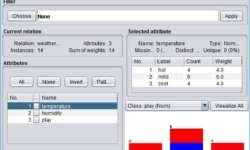This is a follow-up post from previous where we were calculating Naive Bayes prediction on the given data set. This time I want to demonstrate how all this can be implemented using the WEKA application. I highly recommend visiting their website and getting the latest release. WEKA is are a compelling machine learning software written in Java. You can find plenty of tutorials on youtube on how to get started with WEKA. So I won’t get into details. I’m sure you’ll be able to follow anyway.

## Simple explanation of Naive Bayes classifier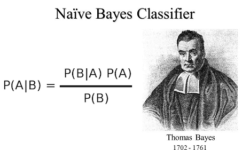Probably you’ve heard about Naive Bayes classifier and likely used in some GUI based classifiers like WEKA package. This is a number one algorithm used to see the initial results of classification. Sometimes surprisingly it outperforms the other models with speed, accuracy and simplicity. Lets see how this algorithm looks and what does it do. As you may know algorithm works on Bayes theorem of probability which allows to predict the class of unknown data set. Hoe you are comfortable with probability math – at least some basics.

## Linear regression with multiple features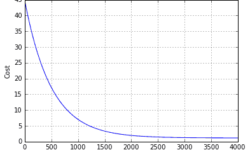Single feature linear regression is simple. All you need is to find a function that fits training data best. It is also easy to plot data and learning curve. But in reality regression analysis is based on multiple features. So in most cases, we cannot imagine the multidimensional space where data could be plotted. We need to rely on the methods we use. You have to feel comfortable with linear algebra where matrices and vectors are used. If previously we had one feature (temperature) now we need to introduce more of them. So we need to expand hypothesis to accept more features. From now and later on instead of output y we are going to use h(x) notation: As you can see with more variables (features), we also end up with more parameters θ that has to be learned. Before we move let’s find suitable data that we could use for building machine learning algorithm. The data set Again we are going to use data set college cengage. This time we select health data set with several variables. The data (X1, X2, X3, X4, X5) are by city. X1 = death rate per 1000 residents X2 = doctor availability per…

## Linear regression – learning algorithm with Python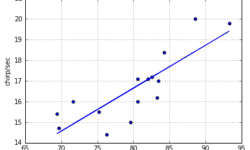In this post, we are going to demystify the learning algorithm of linear regression. We are going to analyze the simplest univariate case with single feature X wherein the previous example was temperature and output was cricket chirps/sec. Let’s use the same data with crickets to build learning algorithm and see if it produces a similar hypothesis as in excel. As you may already know from this example, we need to find linear equation parameters θ0 and θ1, to fit line most optimally on the given data set: y = θ0 + θ1 x x here is a feature (temperature) and y – output value (chirps/sec). So how we are going to find parameters θ0 and θ1? The whole point of the learning algorithm is doing this iteratively. We need to find optimal θ0, θ1 parameter values, so that approximation line error from the plotted training set is minimal. By doing successive corrections to randomly selected parameters we can find an optimal solution. From statistics, you probably know the Least Mean Square (LMS) algorithm. It uses gradient-based method of steepest descent.

## Simplest machine learning algorithm – linear regression with excel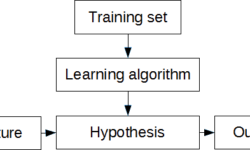Some may say that linear regression is more statistical problem. And this is truth at some level. But when problem is solved from machine learning perspective, things gets easier especially when moving towards more complex problems. First of all lets understand few important terms. We can start with regression. When speaking of linear regression we try to find best fitting line through given points. In other words we need to find optimal linear equation to fit given data points. This is a supervised learning problem when we have set of data pairs that can be plot on x-y axis. I understand, theory is boring thing, even for me, so lets move to practical example and learn by solving some problem. In order to work with some examples we need sample data. There are many data sources available on internet. For instance great source is college cengage that have several sets with data pairs meant for linear regression problems. For our example we are going to use Cricket Chirps Vs. Temperature data where each data point consists of chirps/sec and temperature in degrees Fahrenheit. You can send data in three formats: excel, mtp and ascii. Lets download excel data where we can…

## Overview of machine learning algorithmsA few years ago, machine learning caught my attention, and since then, my interest in this field keeps growing. Every day we see more and more intelligent solutions surrounding us. You probably noticed that shopping sites adapt to our interests and suggests targeted offers; another example is spam email filters; if we mark emails as spam, they keep disappearing from our lives. Another area is robotics, where they learn how to navigate independently and perform various tasks. Machine learning algorithms cover autonomous flying robots, helicopters, quads, handwriting recognition, computer vision, data mining, and multiple fields like markets, biomedicine, biology – all this.  Here are a few reasons why machine learning is significant and sometimes necessary: Data mining. Sometimes it isn’t possible to understand the nature data and relations between them so that machine learning algorithms can extract these hidden relations. Adaptation. It is hard to design a flexible algorithm that could adapt to a changing environment. Machine learning algorithms can be used to improve themselves according to changing data. Scale. There can be much knowledge in data sets that sometimes aren’t completely understood by a human designer. Machine learning algorithms can learn from this knowledge without human interaction. Complexity. The…